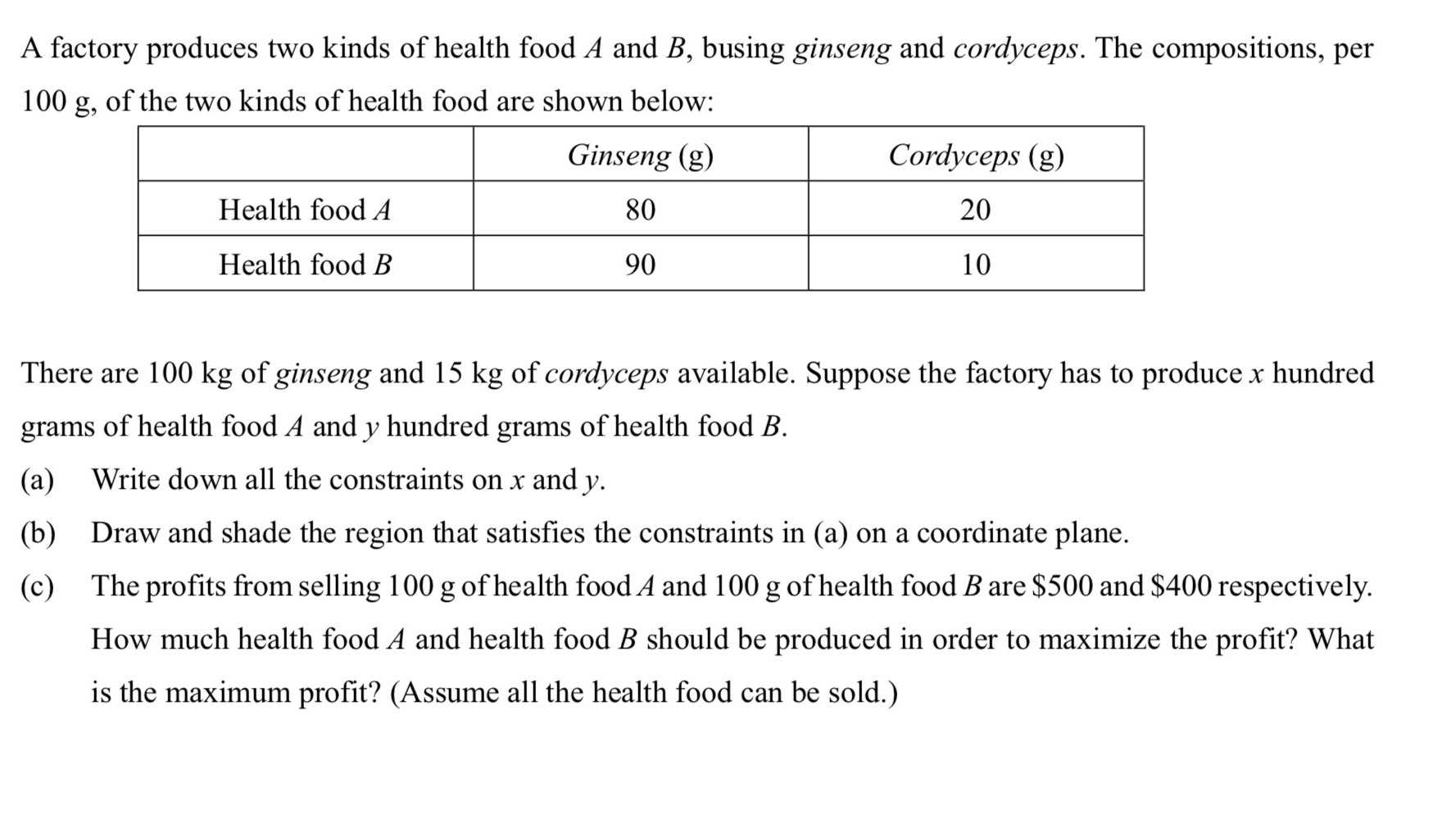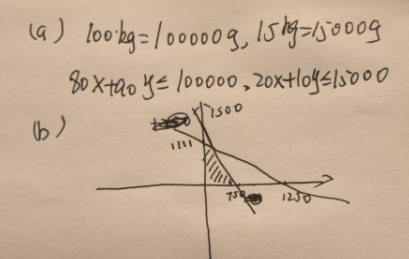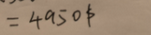### ¿Todavía tienes preguntas de matemáticas?

Pregunte a nuestros tutores expertos
Algebra
PreguntaA factory produces two kinds of health food $$A$$ and $$B$$ , busing ginseng and cordyceps. The compositions, per $$100 g$$ , of the two kinds of health food are shown below:

There are $$100 kg$$ of ginseng and $$15 kg$$ of cordyceps available. Suppose the factory has to produce $$x$$ hundred grams of health food $$A$$ and $$y$$ hundred grams of health food $$B$$ .

(a) Write down all the constraints on $$x$$ and $$y$$ .

(b) Draw and shade the region that satisfies the constraints in (a) on a coordinate plane.

(c) The profits from selling $$100$$ g of health food $$A$$ and $$100$$ g of health food $$B$$ are $$\ 500$$ and $$\ 400$$ respectively. How much health food $$A$$ and health food $$B$$ should be produced in order to maximize the profit? What is the maximum profit? (Assume all the health food can be sold.)